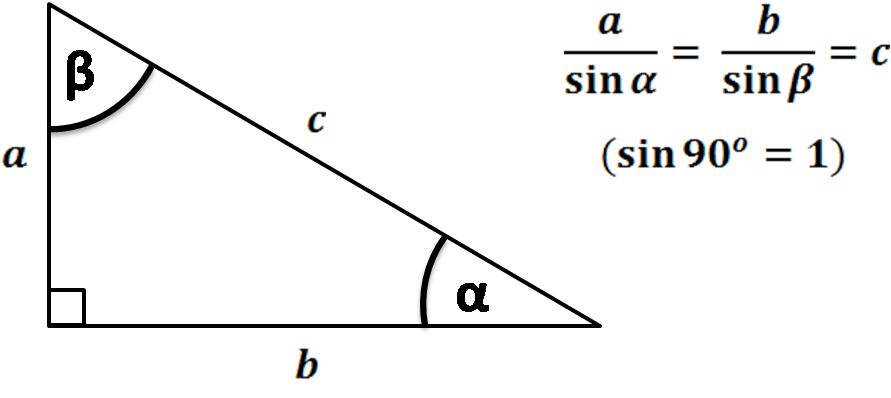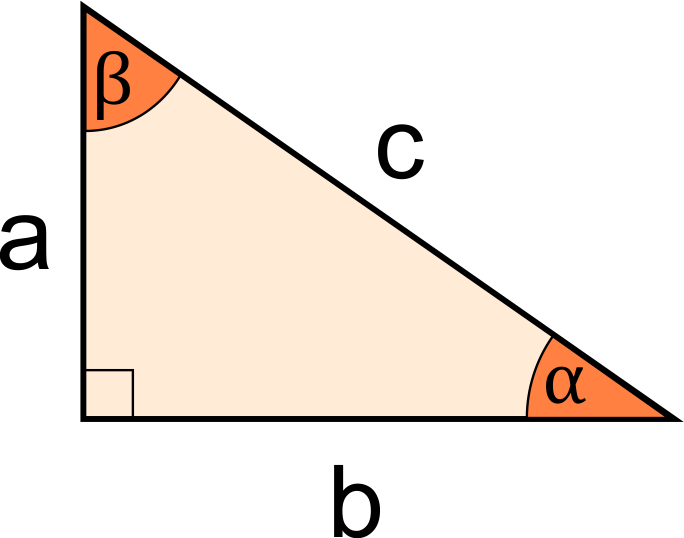# Right Triangle Side and Angle Calculator

Created by Hanna Pamuła, PhD
Reviewed by Bogna Szyk and Jack Bowater
Last updated: Nov 18, 2022

Finding the missing side or angle couldn't be easier than with our great tool – right triangle side and angle calculator. Choose two given values, type them into the calculator, and the calculator will determine the remaining unknowns in a blink of an eye! If you are wondering how to find the missing side of a right triangle, keep scrolling, and you'll find the formulas behind our calculator.

## How to find the sides of a right triangle

There are a few methods of obtaining right triangle side lengths. Depending on what is given, you can use different relationships or laws to find the missing side:

1. Given two sides

If you know two other sides of the right triangle, it's the easiest option; all you need to do is apply the Pythagorean theorem:

a² + b² = c²

• If leg a is the missing side, then transform the equation to the form where a is on one side and take a square root:

a = √(c² - b²)

• If leg b is unknown, then:

b = √(c² - a²)

• For hypotenuse c missing, the formula is:

c = √(a² + b²)

🙋 Our Pythagorean theorem calculator will help you if you have any doubts at this point.

2. Given an angle and the hypotenuseApply the law of sines or trigonometry to find the right triangle side lengths:

• a = c × sin(α) or a = c × cos(β)

• b = c × sin(β) or b = c × cos(α)

🙋 Refresh your knowledge with Omni's law of sines calculator!

3. Given an angle and one leg

Find the missing leg using trigonometric functions:

• a = b × tan(α)

• b = a × tan(β)

4. Given the area and one leg

As we remember from basic triangle area formula, we can calculate the area by multiplying the triangle height and base and dividing the result by two. A right triangle is a special case of a scalene triangle, in which one leg is the height when the second leg is the base, so the equation gets simplified to:

area = a × b / 2

For example, if we know only the right triangle area and the length of the leg a, we can derive the equation for the other sides:

• b = 2 × area / a
• c = √(a² + (2 × area / a)²)

🙋 For this type of problem, see also our area of a right triangle calculator.

## How to find the angle of a right triangle

If you know one angle apart from the right angle, the calculation of the third one is a piece of cake:

Given β: α = 90 - β

Given α: β = 90 - α

However, if only two sides of a triangle are given, finding the angles of a right triangle requires applying some basic trigonometric functions:

for α:

• sin(α) = a / c so α = arcsin(a / c) (inverse sine);
• cos(α) = b / c so α = arccos(b / c) (inverse cosine);
• tan(α) = a / b so α = arctan(a / b) (inverse tangent);
• cot(α) = b / a so α = arccot(b / a) (inverse cotangent);

and for β:

• sin(β) = b / c so β = arcsin(b / c) (inverse sine);
• cos(β) = a / c so β = arccos(a / c) (inverse cosine);
• tan(β) = b / a so β = arctan(b / a) (inverse tangent);
• cot(β) = a / b so β = arccot(a / b) (inverse cotangent).

## How do you solve a right angle triangle with only one side?

To solve a triangle with one side, you also need one of the non-right angled angles. If not, it is impossible:

1. If you have the hypotenuse, multiply it by sin(θ) to get the length of the side opposite to the angle.

2. Alternatively, multiply the hypotenuse by cos(θ) to get the side adjacent to the angle.

3. If you have the non-hypotenuse side adjacent to the angle, divide it by cos(θ) to get the length of the hypotenuse.

4. Alternatively, multiply this length by tan(θ) to get the length of the side opposite to the angle.

5. If you have an angle and the side opposite to it, you can divide the side length by sin(θ) to get the hypotenuse.

6. Alternatively, divide the length by tan(θ) to get the length of the side adjacent to the angle.

## How to find the missing side of a right triangle? How to find the angle? Example

Let's show how to find the sides of a right triangle with this tool:

1. Assume we want to find the missing side given area and one side. Select the proper option from a drop-down list. It's the third one.

2. Type in the given values. For example, an area of a right triangle is equal to 28 in² and b = 9 in.

3. Our right triangle side and angle calculator displays missing sides and angles! Now we know that:

• a = 6.222 in
• c = 10.941 in
• α = 34.66°
• β = 55.34°

Now, let's check how finding the angles of a right triangle works:

1. Refresh the calculator. Pick the option you need. Assume that we have two sides, and we want to find all angles. The default option is the right one.

2. Enter the side lengths. Our right triangle has a hypotenuse equal to 13 in and a leg a = 5 in.

3. Missing side and angles appear. In our example, b = 12 in, α = 67.38° and β = 22.62°.

## FAQ

### How many lines of symmetry does a right triangle have?

If a right triangle is isosceles (i.e., its two non-hypotenuse sides are the same length), it has one line of symmetry. Otherwise, the triangle will have no lines of symmetry.

### Can a right angled triangle have equal sides?

No, a right triangle cannot have all 3 sides equal, as all three angles cannot also be equal. One has to be 90° by definition. A right triangle can, however, have its two non-hypotenuse sides equal in length. This would also mean the two other angles are equal to 45°.

### Are all right triangles similar?

Not all right-angled triangles are similar, although some can be. They are similar if all their angles are the same length, or if the ratio of two of their sides is the same.

Hanna Pamuła, PhD
Given
two sidesa
in
b
in
c
in
Angle α
deg
Angle β
deg
People also viewed…

### Black hole collision

The Black Hole Collision Calculator lets you see the effects of a black hole collision, as well as revealing some of the mysteries of black holes, come on in and enjoy!

### Books vs e-Books